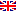Scheda programma d'esame
MODELLI MATEMATICI IN BIOMEDICINA E FISICA MATEMATICA
CYRILL MURATOV
CdSMATEMATICA
Codice559AA
CFU6
PeriodoSecondo semestre
LinguaItaliano

ModuliSettore/iTipoOreDocente/i
MODELLI MATEMATICI IN BIOMEDICINAMAT/05LEZIONI42
 CYRILL MURATOV unimapEsporta in pdf
Programma non disponibile nella lingua selezionata
Learning outcomes
Knowledge

Partial differential equations of mathematical physics and biomedicine, with the emphasis on model derivation from the underlying physical principles, distributional formulation, fundamental solutions in free space, Fourier techniques, separation of variables and special functions. Several examples of nonlinear equations and phenomena associated with nonlinearities will also be discussed.

Syllabus

week 1: PDEs of mathematical physics, conservation laws, equation types, model
derivations

week 2: First order equations and the method of characteristics, classification of
second order quasilinear PDEs

week 3: Distributions in R^N, convolutions, tempered distributions. Fourier transform

week 4: Fundamental solutions for linear differential operators

week 5: Distributional Cauchy problem for the wave equation, wave propagation,
Helmholtz operator

week 6: Distributional Cauchy problem for the heat equation, Newtonian potential

week 7: Elliptic boundary value problems, eigenvalue problems

week 8: Sturm-Liouville problem, Bessel functions, spherical functions

week 9: Initial-boundary value problems

week 10-11: Nonlinear equations and nonlinear phenomena: existence, regularity,
special solutions, blowup

Bibliography

There is no required textbook for this class. The following texts can be used as references:

- Equations of Mathematical Physics, by V. S. Vladimirov, 1985, ISBN 9780828528771
(available in Italian)

- Partial Differential Equations, by J. Kevorkian, 2010, ISBN 9781441931399

- Partial Differential Equations: An Introduction, by W. A. Strauss, 2008,
ISBN 9780470054567

Assessment methods

final oral presentation

Ultimo aggiornamento 21/03/2023 15:26